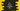# Introduction to Vector containers in C++ STL## Introduction to Vector containers in C++ STL:

We can’t change the size of an array. We have to define the maximum size of the array while initializing the array and we can’t add more than the maximum size. It may throw exception if you try to add more elements than the defined size.

Vectors are dynamic arrays. i.e. it’s size increases automatically if you add new elements. All elements are stored in contiguous memory locations and it allocates new memory automatically.

Similar to arrays, we can access the elements in a vector and we can iterate through a vector easily.

### Iterators in vectors:

Following are the iterators defined in vectors:

• begin() : It returns an iterator that points to the start of the vector
• end() : It returns an iterator that points to the end of the vector
• rbegin() : rbegin or reverse begin returns a reverse iterator that points to the end of the vector.
• rend(): rend or reverse end returns a reverse iterator that points to the theoretical element preceding the 1st element of the vector.

#### Available only on C++11

• cbegin(): It returns a constant iterator that points to the start of the vector.
• cend(): It returns a constant iterator that points to the theoretical element preceding the last vector element.
• crbegin(): It returns a constant reverse iterator that points to the end of the vector.
• crend(): It returns a constant reverse iterator that points to the theoretical element preceding the first vector element.

### Capacity functions in vectors:

• size(): Get the total number of elements in a vector
• max_size: Get the maximum size
• resize: Resize a vector.
• empty: Check if a vector is empty or not
• capacity: Get the currently allocated space of a vector
• reserve: Use this to change the capacity of a vector

#### Available only on C++11

• shrink_to_fit: Shrink the vector to fit its size

### Accessing elements of a vector:

• []: Access an element of a vector by using an index
• at: Access an element of a vector by using an index
• front: Access the start element of a vector.
• back: Access the last element of a vector.

#### Available only on C++11

• data: Get the pointer to the array used by the vector to store the elements.

### Modifiers:

• assign: Assign new content to a vector.
• push_back: Add an element to the end of a vector.
• pop_back: Add an element to the start of a vector.
• insert: Insert element to a vector.
• erase: Erase elements from a vector.
• swap: Swap elements in a vector.
• clear: Clear vector contents.

#### Available only on C++11

• emplace: This is used to construct and insert element to a vector.
• emplace_back: This is used to construct and insert an element to the end of a vector.

### Allocator:

• get_allocator: It returns a copy of the allocator object of the vector.